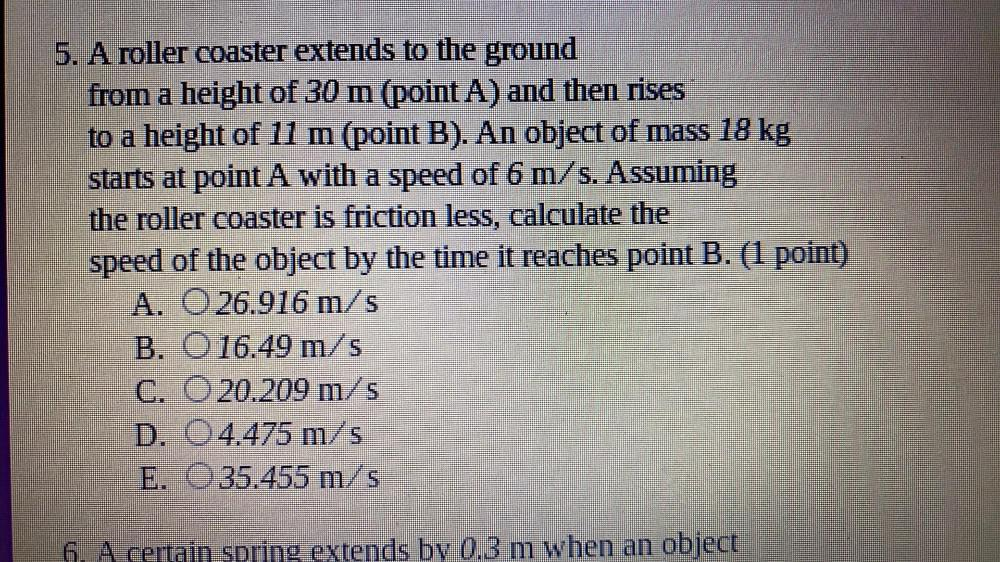Question:

# 5. A roller coaster extends to the ground from a height of 30 m (point A) and then rises to a height of 11 m (point B). An objec5. A roller coaster extends to the ground from a height of 30 m (point A) and then rises to a height of 11 m (point B). An object of mass 18 kg starts at point A with a speed of 6 m/s. Assuming the roller coaster is friction less, calculate the speed of the object by the time it reaches point B. (1 point) A. O 26.916 m/s B. O 16.49 m/s C. O 20.209 m/s D. O 4.475 m/s E. O35.455 m/s 6. A certain spring extends by 0.3 m when an object